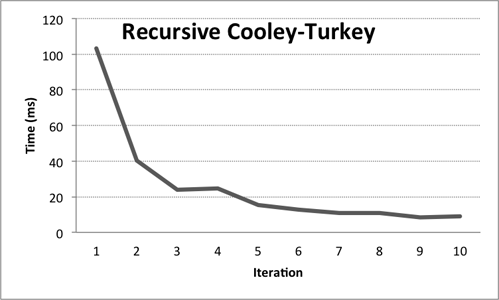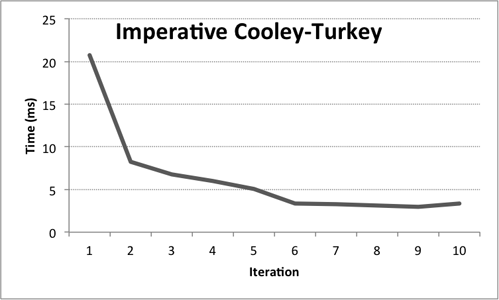# Numerical Computing With Scala

In the previous post, we looked at the Fourier transform function. In this post, we’ll explore some implementations of this function in Scala and capture some performance metrics.

Before we start, we need a data representation for complex numbers and a pure trait to test different FFT functions. Note that the FFT trait specifies a Numeric type class so it can work with any sequence of numbers.

As mentioned in the previous post, the Cooley-Turkey algorithm requires that the data length be a power of 2. All of our equations in the previous post were in terms of the complex exponential function (eix). Using Euler’s formula we can instead rely on sine and cosine functions in our implementations.## Recursive Implementation

The recursive nature of the standard Cooley-Turkey algorithm lends itself nicely to a pure functional implementation. Since we should always prefer pure functional code, we’ll start there.

This actually follows the mathematical definition pretty nicely and is very concise and readable. Note that we are recursively breaking the data into smaller DFTs by even and odd indexes. We’re calculating the phase (twiddle) factors seperately and relying on the symmetric properties of the DFT to recombine the values for 0 ≤ k < N/2 and N/2 ≤ k < N.

So how well does this algorithm perform? First try with 1024 random Double values on my machine takes ~ 100 ms. OK, let’s see how this does once the machine warms up. If we try 10 random sequences in a row (size 1024), we get:We can see that it’s starting to settle. After running 1000 iterations, we get an average of ~ 3 ms per fft call.

## Imperative Implementation

It’s no secret that optimizing Scala code can sometimes be ugly (see Erik Osheim’s Premature Optimization). So let’s see if we move towards an imperative version of the FFT.

The following is basically a translation of the algorithm from Apache Commons-Math into Scala. This algorithm is still based on the Cooley-Turkey algorithm, but the implementation is much more verbose and harder to follow than the recursive version.

Yikes, we went from 30 lines of code to 120. Let’s take a look at a few points in this algorithm though. Since we are no longer recursively selecting even/odd indexes, we perform a bit-reverse shuffle of the data up front. This allows us to traverse the data in essentially the same order. Also, note that the WNk real and imaginary factors are pre-computed. This, in combination with the fact that we are operating on arrays and avoiding boxing and unboxing will certainly make this algorithm faster. Let’s see how much.

Using the same test as before, our first try with 1024 random samples takes ~ 20 ms. Next up, let’s test with the warmup using 10 iterations:After 1000 iterations, it takes an average of ~ 0.19 ms per fft call.

## Conclusions

The following table shows a side-by-side comparison of both algorithms. The times in these tables were averaged from 1000 iterations on increasing frame sizes.

Frame Size Recursive Time (ms) Imperative Time (ms)
512 1.56 0.14
1024 2.98 0.19
2048 6.04 0.27
4096 13.18 0.47

The imperative algorithm is clearly faster, but much more verbose and harder to understand.

Scala gets knocked sometimes for allowing both OO/imperative and functional styles of coding. In my opinion this is actually a huge benefit for the language. You can favor the functional style and resort to imperative code in cases where performance is critical. These cases can be isolated and the details can be hidden. Looking at our imperative algorithm above, the FFT is still referentially transparent.